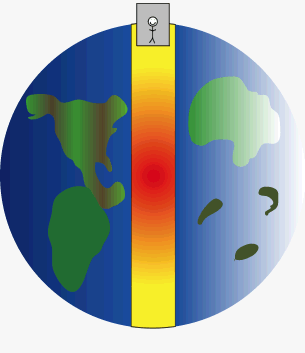Home » Posts tagged 'weightlessness'

# Tag Archives: weightlessness

## Gravity

We all know there is no such thing as zero gravity even at the very end of universe there is micro gravity according to formula for gravity…!
Means we are away from certain object so the gravity exist in vacuum so now my question is:-
Q- We all know that there is zero Gravity in center of earth core so if we place an object in center of earth the would Gravity formula apply on that object or that object is in complete Zero gravity…????

If we simply keep a body at the centre, it will remain there as it is attracted equally in all directions.A man in an elevator free falls through a planet to simulate how a gravity train could work. At both ends of the planet the train comes to a complete stop, but free falls through the middle (or wherever the tunnel is constructed) of the planet. (Photo credit: Wikipedia)

# GRAVITY## Weightlessness in a satellite

Why does an astronaut feel weightless in a space capsule orbiting the earth?

I’ve gotten a key point saying that the space capsule orbiting the earth has a centripetal acceleration, but I cannot figure out why is it related to the question.

Answer: The centripetal acceleration is provided by the force of gravity. Since the force of gravity is completely used up in providing the centripetal acceleration to the space capsule, the astronaut feel weightlessness.## BMMD – Body Mass Measuring Device

What is a BMMD? How does it work?

Ans:

BMMD stands for Body Mass Measuring Device used by astronauts, which is basically a spring mounted chair which measures mass as a measure of inertia. As you know, one experiences weightlessness inside a satellite and normal methods of measuring mass do not work. (like a common balance or a spring balance). The time period of oscillations which depends on the force constant and mass is used in measuring mass in BMMD used by an astronomer

## Time Period of a Simple Pendulum in Space

“if you set up a pendulum aboard an orbiting space vehicle, would the period be less than, the same as, or greater than it would be in our lab? explain.”

Ans:

In a space vehicle orbiting around the earth, the apparent weight is zero as the entire gravitational pull is being utilized in providing the centripetal force required for revolving. This is called weightlessness in space.

i.e; in effect, the acceleration due to gravity is zero which make the time period of the satellite infinity. The pendulum will not oscillate at all.

__________________________________________________________

Tailpiece:

However, in manned space vehicles, there is an option to simulate gravity by making the system rotate at a particular rate so as to make the inhabitants feel as if there is weight and gravity.

### Hits so far @ AskPhysics

• 2,215,154 hits

### CBSE Exam Count Down

AISSCE / AISSE 2020February 26, 2020
Board Exams for the core subjects of CBSE may start from 26 February (Class X) and 27 February (Class XII)